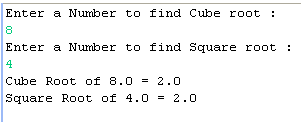Tutorials

# Java Square Root And Cube Root Example

This example explains you about finding the square root and cube root of a number. This tutorial explains about all the steps of how to find the square root and cube root. In this example we will use JDK 1.6 and the Eclipse IDE for writing, compiling and executing the code.

This example explains you about finding the square root and cube root of a number. This tutorial explains about all the steps of how to find the square root and cube root. In this example we will use JDK 1.6 and the Eclipse IDE for writing, compiling and executing the code.

## Java Square Root And Cube Root Example

In this section we will discuss about how to find the square root and cube root of a number.

This example explains you about finding the square root and cube root of a number. This tutorial explains about all the steps of how to find the square root and cube root. In this example we will use JDK 1.6 and the Eclipse IDE for writing, compiling and executing the code.

Example

Here an example is being given which will demonstrate you about finding out the square root and cube root of a number. In this example we will first create a Java project in Eclipse and then we will create a package inside the src folder and then we will create a Java class for finding out the square root and cube root of a number. In this example we will read the number, which roots we have to find, from the command line.

Source Code

RootExample.java

```package desktop;

import java.io.*;
public class CubeRootExample {

public static void main(String args[])
{
try
{
System.out.println("Enter a Number to find Cube root : ");
System.out.println("Enter a Number to find Square root : ");

double cubeResult = Math.pow(cube, 1.0/3.0);
System.out.println("Cube Root of "+cube+" = "+cubeResult);

double squareResult = Math.pow(square, 1.0/2.0);
System.out.println("Square Root of "+square+" = "+squareResult);
}
catch(Exception e)
{
System.out.println(e);
}
}
}
```

Output

To execute the above code you can use the Ctrl+F11 in Eclipse.

After successfully execution of the above code at console a message will be prompt for input the number for finding Cube Root and when you will press Enter an another message will be prompt to input the number for finding the square root and after input both the number when you will press Enter then the result will be displayed as follows :